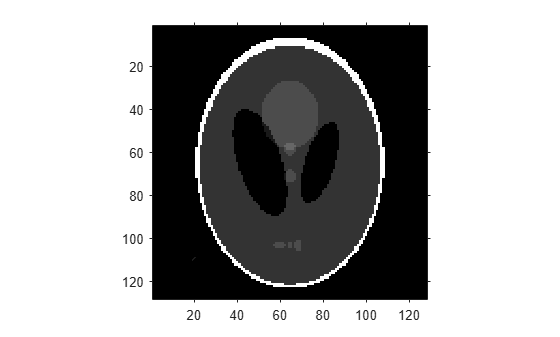# fanbeam

Fan-beam transform

## Syntax

``F = fanbeam(I,D)``
``F = fanbeam(I,D,Name,Value)``
``````[F,fanSensorPos,fanRotAngles] = fanbeam(___)``````

## Description

example

````F = fanbeam(I,D)` calculates the fan-beam projection data (sinogram) `F` from the image `I`. Each column of `F` contains fan-beam projection data at one rotation angle. `D` is the distance from the fan-beam vertex to the center of rotation.```
````F = fanbeam(I,D,Name,Value)` uses name-value arguments to specify the rotation increment and sensor spacing.```

example

``````[F,fanSensorPos,fanRotAngles] = fanbeam(___)``` also returns the location of fan-beam sensors in `fanSensorPos` and the rotation angles where the fan-beam projections are calculated in `fanRotAngles`.```

## Examples

collapse all

Set the IPT preference to make the axes visible.

`iptsetpref('ImshowAxesVisible','on')`

Create a sample image and display it.

```ph = phantom(128); imshow(ph)```Calculate the fanbeam projections and display them.

```[F,Fpos,Fangles] = fanbeam(ph,250); figure imshow(F,[],'XData',Fangles,'YData',Fpos,... 'InitialMagnification','fit') axis normal xlabel('Rotation Angles (degrees)') ylabel('Sensor Positions (degrees)') colormap(gca,hot), colorbar```Compute fan-beam projections for 'arc' geometry.

```I = ones(100); D = 200; dtheta = 45; [Farc,FposArcDeg,Fangles] = fanbeam(I,D,... 'FanSensorGeometry','arc',... 'FanRotationIncrement',dtheta);```

Convert angular positions to linear distance along x-prime axis.

`FposArc = D*tan(FposArcDeg*pi/180);`

Compute fan-beam projections for 'line' geometry.

```[Fline,FposLine] = fanbeam(I,D,... 'FanSensorGeometry','line',... 'FanRotationIncrement',dtheta);```

`[R,Rpos]=radon(I,Fangles);`

Display the three projections at one particular rotation angle. Note the three are very similar. Differences are due to the geometry of the sampling, and the numerical approximations used in the calculations.

```figure idx = find(Fangles==45); plot(Rpos,R(:,idx),... FposArc,Farc(:,idx),... FposLine,Fline(:,idx)) legend('Radon','Arc','Line')```## Input Arguments

collapse all

Input image, specified as a 2-D numeric matrix or 2-D logical matrix.

Distance in pixels from the fan beam vertex to the center of rotation, specified as a positive number. The center of rotation is the center pixel of the image, defined as `floor((size(I)+1)/2)`. `D` must be large enough to ensure that the fan-beam vertex is outside of the image at all rotation angles. See Tips for guidelines on specifying `D`.### Name-Value Arguments

Specify optional pairs of arguments as `Name1=Value1,...,NameN=ValueN`, where `Name` is the argument name and `Value` is the corresponding value. Name-value arguments must appear after other arguments, but the order of the pairs does not matter.

Example: `fanbeam(I,D,FanRotationIncrement=5)`

Before R2021a, use commas to separate each name and value, and enclose `Name` in quotes.

Example: `fanbeam(I,D,"FanRotationIncrement",5)`

Fan-beam rotation angle increment in degrees, specified as a positive scalar.Data Types: `double`

Fan-beam sensor positioning, specified as `"arc"` or `"line"`.

Value

Meaning

Diagram

`"arc"`

Sensors are spaced at equal angles along a circular arc. The center of the arc is the fan-beam vertex.

`FanSensorSpacing` defines the angular spacing in degrees.`"line"`

Sensors are spaced at equal distances along a line that is parallel to the x' axis. The closest sensor is distance `D` from the center of rotation.

`FanSensorSpacing` defines the distance between fan-beams on the x' axis, in pixels.Fan-bean sensor spacing, specified as a positive scalar.

• If `FanSensorGeometry` is `"arc"`, then `FanSensorSpacing` defines the angular spacing in degrees.

• If `FanSensorGeometry` is `"line"`, then `FanSensorSpacing` defines the linear distance between fan-beams, in pixels. Linear spacing is measured on the x' axis.

Data Types: `double`

## Output Arguments

collapse all

Fan-beam projection data, returned as a numSensors-by-numAngles numeric matrix. numSensors is the number of fan-beam sensors and numAngles is the number of fan-beam rotation angles. Each column of `F` contains the fan-beam sensor samples at one rotation angle.

`fanbeam` determines numAngles by calculating how many rotation angles are required to span 360 degrees using an angular spacing of `FanRotationIncrement`.

`fanbeam` determines numSensors by calculating how many beams are required to cover the entire image for any rotation angle. Fewer sensors are required to cover the image when the distance `D` between the fan-beam vertex and the center of rotation is large.

Data Types: `double`

Location of fan-beam sensors, returned as a numSensors-by-1 numeric vector.

• If `FanSensorGeometry` is `"arc"` (the default), then `fanSensorPos` contains the fan-beam spread angles.

• If `FanSensorGeometry` is `"line"`, then `fanSensorPos` contains the fan-beam sensor positions along the x' axis. See `FanSensorSpacing` for more information.

Data Types: `double`

Rotation angle of fan-beam sensors, returned as a 1-by-numAngles numeric vector.

Data Types: `double`

## Tips

As a guideline, try making `D` a few pixels larger than half the image diagonal dimension, calculated as follows.

`sqrt(size(I,1)^2 + size(I,2)^2)`

The values returned in `F` are a numerical approximation of the fan-beam projections. The algorithm depends on the Radon transform, interpolated to the fan-beam geometry. The results vary depending on the parameters used. You can expect more accurate results when the image is larger, `D` is larger, and for points closer to the middle of the image, away from the edges.

 Kak, Avinash C., and Malcolm Slaney. Principles of Computerized Tomographic Imaging. New York: IEEE Press, 1988.. pp. 92-93.